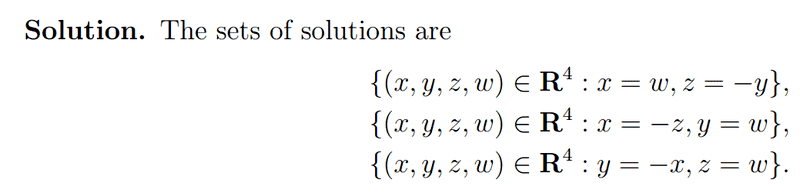# How is it possible to solve this system of 2 equations with 4 unknowns?

jdinatale
I've always been taught that to solve for 4 unknowns, you need 4 equations. This question seems to suggest that there are in fact solutions. Here's the problem and my work so far. The most I can seem to do is eliminate one variable, I'm not sure how to shake a second one out, let alone all 4.Homework Helper
Gold Member
I've always been taught that to solve for 4 unknowns, you need 4 equations.
For linear equations: You need 4 equations in order for there to be a UNIQUE solution. With fewer equations than unknowns, there will be either infinitely many solutions or no solutions, depending on whether the equations are consistent or not.

However, note that your second equation is not linear in x, y, and z.

Your work so far is correct, but I don't think it's getting you any closer to a solution.

I suggest solving one of the equations for one of the variables in terms of the others. Then substitute into the other equation and simplify. Most likely there will be infinitely many solutions, so the goal is to try to characterize the set of solutions in the simplest way possible.

Staff Emeritus
Homework Helper
If you have four unknowns, you need four independent equations to find a unique solution. You won't be able to find a unique solution in this case.

jdinatale
Well, I've found a fairly trivial set of solutions, but are these the only ones?

Solutions 1: {x = w, z = -y}
Solution 2: {x = -z, y = w}
Solution 3: {y = x, z = w}

The real question is - are these solutions the only ones?

Homework Helper
Gold Member
Actually this equation might be on the right track:

$$yz + xz + xy = \frac{xyz}{x + y + z}$$

Try solving for one of the variables in terms of the others. This will probably involve the quadratic formula. Also be careful that this equation is true only if $x + y + z \neq 0$.

jdinatale
Actually this equation might be on the right track:

$$yz + xz + xy = \frac{xyz}{x + y + z}$$

Try solving for one of the variables in terms of the others. This will probably involve the quadratic formula. Also be careful that this equation is true only if $x + y + z \neq 0$.

I think we're off track. I've confirmed using mathematica thatwhich is true for all x + y + z /= 0 and y + z /= 0.

However, this is a putnam problem, so I know that this isn't the sort of solution they are looking for. There has to be a more elegant solution.

Staff Emeritus